# Class 8 RD Sharma Solutions – Chapter 17 Understanding Shapes Special Types Of Quadrilaterals – Exercise 17.2 | Set 2

### Question 9. One side of a rhombus is of length 4 cm and the length of an altitude is 3.2 cm. Draw the rhombus.

Solution: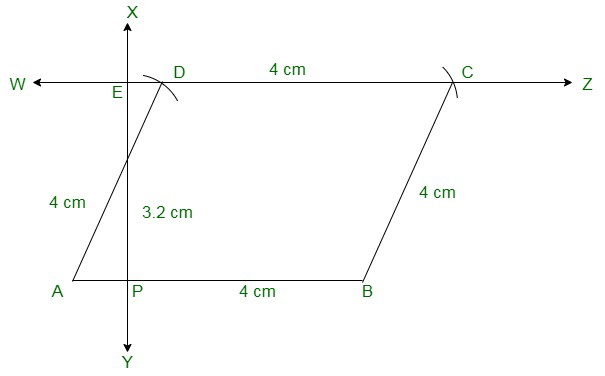Steps to construct a rhombus:

(i) Draw a line segment of 4 cm

(ii) From point A draw a perpendicular line bisecting the length of 3.2 cm to get point E.

(iii) From point E draw a line parallel to AB.

(iv) From points A and B cut two arcs of length 4 cm on the drawn parallel line to get points D and C.

(v) Join the line segments AD, BC and CD to get rhombus ABCD.

### Question 10. Draw a rhombus ABCD, if AB = 6 cm and AC = 5 cm.

Solution: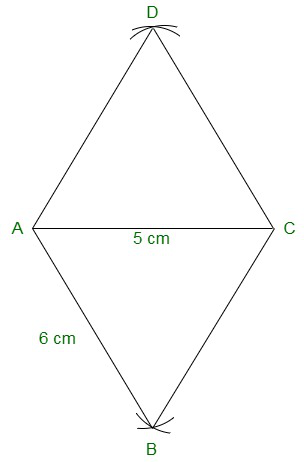Steps of construction:

(i) Draw a line segment AB of length 6 cm.

(ii) From point ‘A’ cut an arc of length 5 cm and from point B cut an arc of length 6 cm intersecting at ‘C’.

(iii) Join the line segments AC and BC.

(iv) From point A cut an arc of length 6 cm and from point C cut an arc of 6cm, so that both the arcs intersect at point D.

(v) Join the remaining line segments AD and DC to get rhombus ABCD.

### Question 11. ABCD is a rhombus and its diagonals intersect at O.

(i) is ΔBOC ≅ ΔDOC? State the congruence condition used?

(ii) Also state, if ∠BCO = ∠DCO.

Solution: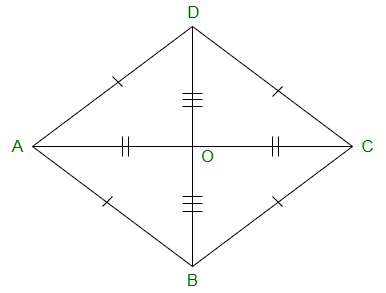(i) Yes,

In ΔBOC and ΔDOC

Since, in a rhombus diagonals bisect each other, we have,

BO = DO

CO = CO Common

BC = CD [All sides of a rhombus are equal]

Now,

By using SSS Congruency, ΔBOC≅ΔDOC

(ii) Yes.

Since by,

∠BCO = ∠DCO, by corresponding parts of congruent triangles.

### Question 12. Show that each diagonal of a rhombus bisects the angle through which it passes.

Solution: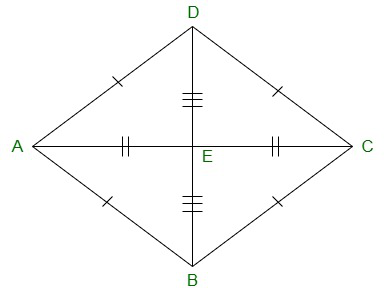(i) In ΔBOC and ΔDOC

BO = DO [In a rhombus diagonals bisect each other]

CO = CO Common

BC = CD [All sides of a rhombus are equal]

By using SSS Congruency, ΔBOC≅ΔDOC

∠BCO = ∠DCO, by corresponding parts of congruent triangles

Therefore,

Each diagonal of a rhombus bisect the angle through which it passes.

### Question 13. ABCD is a rhombus whose diagonals intersect at O. If AB=10 cm, diagonal BD = 16 cm, find the length of diagonal AC.

Solution: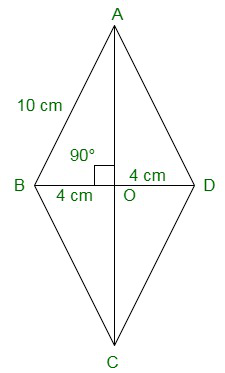In a rhombus diagonals bisect each other at right angle.

In ΔAOB

BO = BD/2 = 16/2 = 8cm

AB2 = AO2 + BO2 (Pythagoras theorem)

102 = AO2 + 82

100-64 = AO2

AO2 = 36

AO = √36 = 6cm

Hence, Length of the diagonal AC is 6 × 2 = 12cm.

### Question 14. The diagonal of a quadrilateral are of lengths 6 cm and 8 cm. If the diagonals bisect each other at right angles, what is the length of each side of the quadrilateral?

Solution: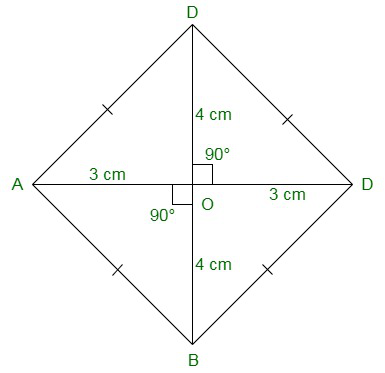In a rhombus diagonals bisect each other at right angle.

Considering ΔAOB

BO = BD/2 = 6/2 = 3cm

AO = AC/2 = 8/2 = 4cm

Now,

AB2 = AO2 + BO2 (Pythagoras theorem)

AB2 = 42 + 32

AB2 = 16 + 9

AB2 = 25

AB = √25 = 5cm

Hence, Length of each side of the quadrilateral ABCD is 5cm.

Whether you're preparing for your first job interview or aiming to upskill in this ever-evolving tech landscape, GeeksforGeeks Courses are your key to success. We provide top-quality content at affordable prices, all geared towards accelerating your growth in a time-bound manner. Join the millions we've already empowered, and we're here to do the same for you. Don't miss out - check it out now!

Previous
Next